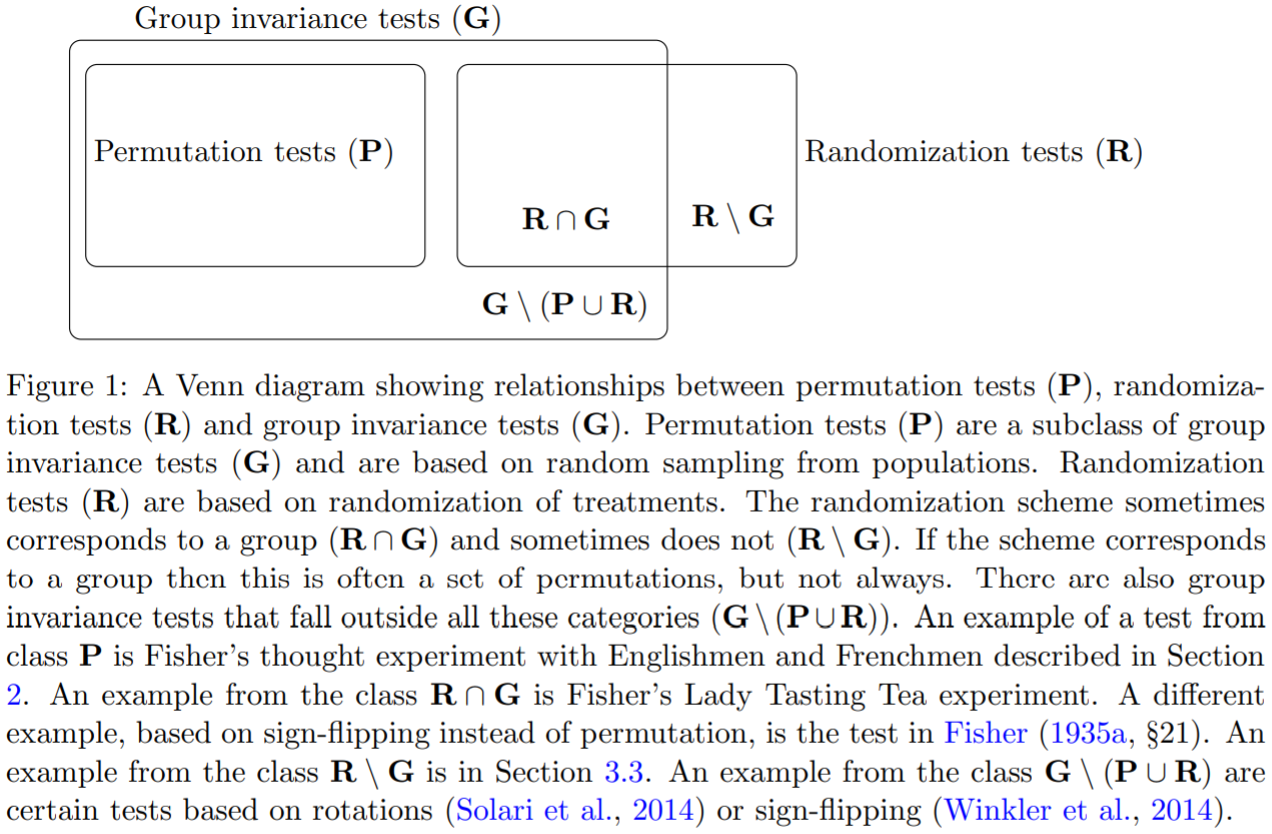# Permutation Tests and Randomization Tests

##### Posted on May 22, 20210 Comments## Group Invariance Test

A general definition of a group invariance test. Let $X$ model random data with support $\cX$. Consider a set $\cG$ of permutation maps or other transformations $g:\cX\rightarrow\cX$. The set $\cG$ is assumed to have a group structure w.r.t. the operation of composition of maps, which means that:

• $\cG$ contains the identity map $x\mapsto x$
• every element in $\cG$ has an inverse
• for all $g,h\in\cG$, $g\circ h\in\cG$.

no association?

Consider some test statistic $T:\cX\rightarrow \IR$.

Consider a null hypothesis $H_0$ that implies that the joint distribution of all test statistics $T(g(X))$ with $g\in \cG$ is invariant under all transformations of $X$ in $\cG$. This holds in particular if the data $X$ are themselves transformation-invariant, i.e., if

$g(X)\overset{d}{=} X$

for every $g\in \cG$.

Let $X_1,\ldots, X_{100}$ be the statures of the Englishmen and let $X_{101},\ldots, X_{200}$ be the statures of the Frenchmen. The test statistic is $$T(X) = \left\vert \frac{1}{100}\sum_{i=1}^{100}X_i-\frac{1}{100}\sum_{i=101}^{200}X_i\right\vert\,.$$ The null hypothesis $H_0$ is that $X_i$’s are i.i.d. The group $\cG$ that Fisher consider consists of all permutation maps $g:\IR^{200}\rightarrow\IR^{200}$. Note that $X$ is $\cG$-invariant under $H_0$.

Consider random data $X$ with support in $\IR^n$, with independent entries that are symmetric about their means. Suppose that under $H_0$, the entries have mean 0. Then the distribution of $X$ is invariant under all transformations in $\cG$ under $H_0$ if we define $\cG$ to be the group of all sign-flipping maps of the form $$(x_1,\ldots, x_n)\mapsto (s_1x_1,\ldots, s_nx_n)\,,$$ with $(s_1,\ldots,s_n)\in{-1,1}^n$. We may take $T((x_1,\ldots,x_n))=\sum_{i=1}^nx_i$.

## Randomization Test

Unlike a permutation test, a randomization test is based on data collected in an experiment involving randomization of treatments.

For the Lady Tasting Tea experiment(refer to a Chinese blog), let $W\subset\{0,1\}^8$ be the set of vectors containing four 0s and four 1s, so that the cardinality of $W$ is $R:=\vert W\vert=70$. Let the decision of the lady be denoted by $Y$ and let $W$ denote the true order. The null hypothesis is $$H_0: Y \text{ is independent of } W\,.$$ Let $\alpha\in(0, 1)$ be the desired type I error rate. If $\alpha\in A=\{1/70, 17/70, 53/70, 69/70\}$, then $\alpha$ is called attainable in the Lady Tasting Tea experiment.

Let $T:W\times W\rightarrow \IR$ be a test statistic such that high values of $T(w, y)$ indicate that the patterns $w$ and $y$ are similar, i.e., that there is evidence against $H_0$. Let $$T^{(1)}(Y)\le \cdots \le T^{(70)}(Y)$$ be the sorted statistics $T(w,Y)$ with $w\in W$. The test statistic is $$T(W, Y)=\sum_{i=1}^81_{\{W_i=1\}\cap\{Y_i=1\}}=\sum_{i=1}^8W_iY_i$$

The test that rejects $H_0$ iff $T(W, Y) > T^{(\lceil (1-\alpha)R\rceil)}$ has size at most $\alpha$.

## Discussion

The paper distinguished between two types of permutation-based tests:

• tests that fundamentally rely on a group structure
• tests based on treatment randomization, which do not necessarily require a group structure

Published in categories Note